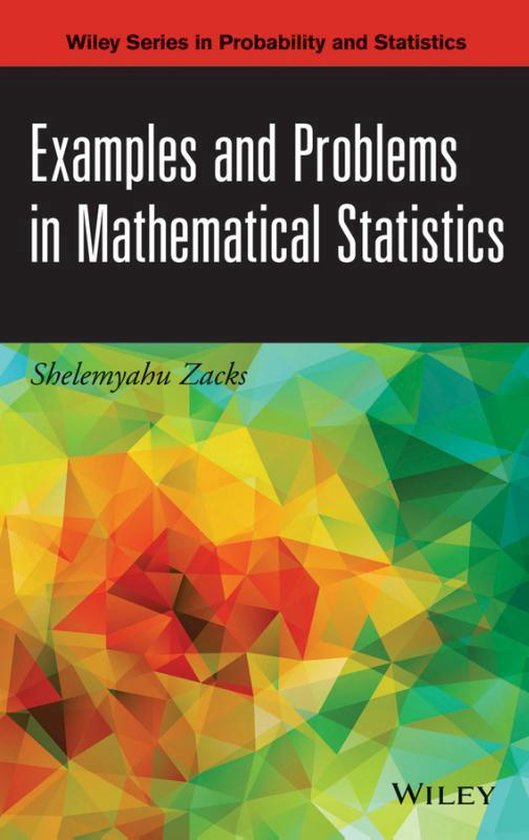Wat is dit voor site? Wij zoeken de goedkoopste boeken op internet. Examples and Problems in Mathematical Statisticsvonden we het goedkoopst op Bol.com voor €156.99. We hebben gezocht in nieuwe en tweedehands boeken. Rechtsonderaan de pagina kan je het boek direct bestellen via Bol .com.

# Examples and Problems in Mathematical Statistics

Engels | Hardcover | 9781118605509 | 652 pagina's

Zacks - 9781118605509

Engels | Hardcover | 9781118605509 | 652 pagina'sProvides the necessary skills to solve problems in mathematical statistics through theory, concrete examples, and exercises With a clear and detailed approach to the fundamentals of statistical theory, Examples and Problems in Mathematical Statistics uniquely bridges the gap between theory andapplication and presents numerous problem-solving examples that illustrate the relatednotations and proven results. Written by an established authority in probability and mathematical statistics, each chapter begins with a theoretical presentation to introduce both the topic and the important results in an effort to aid in overall comprehension. Examples are then provided, followed by problems, and finally, solutions to some of the earlier problems. In addition, Examples and Problems in Mathematical Statistics features: * Over 160 practical and interesting real-world examples from a variety of fields including engineering, mathematics, and statistics to help readers become proficient in theoretical problem solving * More than 430 unique exercises with select solutions * Key statistical inference topics, such as probability theory, statistical distributions, sufficient statistics, information in samples, testing statistical hypotheses, statistical estimation, confidence and tolerance intervals, large sample theory, and Bayesian analysis Recommended for graduate-level courses in probability and statistical inference, Examples and Problems in Mathematical Statistics is also an ideal reference for applied statisticians and researchers.

Levertijd:   Verwacht over 7 weken

156.99

Examples and Problems in Mathematical Statistics Engels | Hardcover | 9781118605509 | 652 pagina's
TaalEngels
BindwijzeHardcover
Verschijningsdatumapril 2014
Aantal pagina's652 pagina's
IllustratiesNee
Auteur(s)Zacks
UitgeverJohn Wiley & Sons Inc
Extra groot lettertypeNee
Gewicht1021 g
StudieboekNee
Verpakking breedte165 mm
Verpakking hoogte33 mm
Verpakking lengte236 mm

Laat hier je e-mail adres achter en de prijs die je voor het boek wil betalen. Wij laten je dan automatisch weten wanneer het boek voor jouw prijs beschikbaar is.